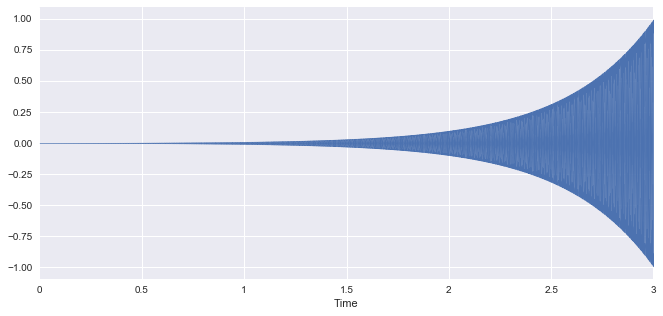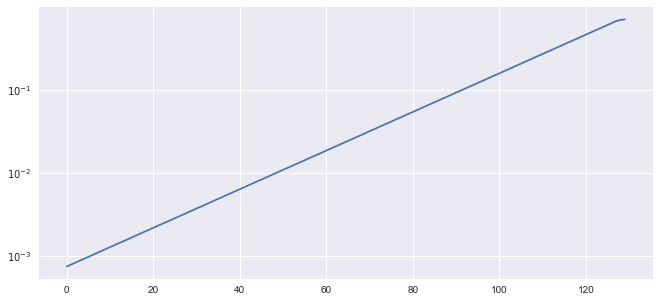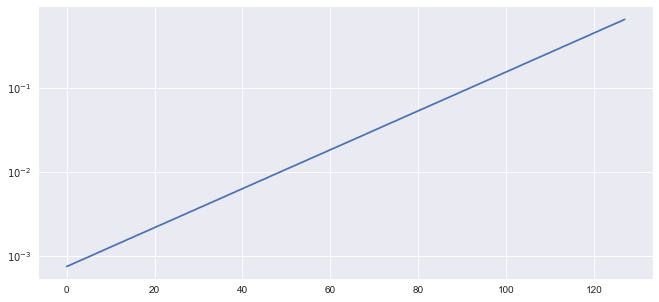In :
%matplotlib inline
import seaborn
import numpy, scipy, matplotlib.pyplot as plt, IPython.display as ipd
import librosa, librosa.display
plt.rcParams['figure.figsize'] = (11, 5)


# SegmentationÂ¶

In audio processing, it is common to operate on one frame at a time using a constant frame size and hop size (i.e. increment). Frames are typically chosen to be 10 to 100 ms in duration.

Let's create an audio signal consisting of a pure tone that gradually gets louder. Then, we will segment the signal and compute the root mean square (RMS) energy for each frame.

First, set our parameters:

In :
T = 3.0      # duration in seconds
sr = 22050   # sampling rate in Hertz
amplitude = numpy.logspace(-3, 0, int(T*sr), endpoint=False, base=10.0) # time-varying amplitude
print amplitude.min(), amplitude.max() # starts at 110 Hz, ends at 880 Hz

0.001 0.999895579824


Create the signal:

In :
t = numpy.linspace(0, T, int(T*sr), endpoint=False)
x = amplitude*numpy.sin(2*numpy.pi*440*t)


Listen to the signal:

In :
ipd.Audio(x, rate=sr)

Out:

Plot the signal:

In :
librosa.display.waveplot(x, sr=sr)

Out:
<matplotlib.collections.PolyCollection at 0x1108b6690>## Segmentation Using Python List ComprehensionsÂ¶

In Python, you can use a standard list comprehension to perform segmentation of a signal and compute RMSE at the same time.

Initialize segmentation parameters:

In :
frame_length = 1024
hop_length = 512


Define a helper function:

In :
def rmse(x):
return numpy.sqrt(numpy.mean(x**2))


Using a list comprehension, plot the RMSE for each frame on a log-y axis:

In :
plt.semilogy([rmse(x[i:i+frame_length])
for i in range(0, len(x), hop_length)])

Out:
[<matplotlib.lines.Line2D at 0x1109bca90>]## librosa.util.frameÂ¶

Given a signal, librosa.util.frame will produce a list of uniformly sized frames:

In :
frames = librosa.util.frame(x, frame_length=frame_length, hop_length=hop_length)
plt.semilogy([rmse(frame) for frame in frames.T])

Out:
[<matplotlib.lines.Line2D at 0x110d36e10>]That being said, in librosa, manual segmentation of a signal is often unnecessary, because the feature extraction methods themselves do segmentation for you.